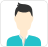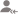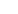true

Find the best tutors and institutes for MATLAB

Find Best MATLAB Training

No matching category found.

No matching Locality found.

Outside India?

Search for topicsPost a Lesson

All

All

Lessons

Discussion

Lesson Posted on 08/11/2018 IT Courses/MATLABManibhushan Kumar

I have 15 years of experience in Maths and Stats. I have started teaching one year after completing my...

A trigonometric identity is given by: cos^2(x/2)=(tanx+sinx)/2tanx We would calculate each side of the equation, substituting.x=pi/2 sol. >> x=pi/5; LHS=cos(x/2)^2 LHS = 0.9045 RHS=(tan(x)+sin(x))/(2*tan(x)) RHS = 0.9045 read more

A trigonometric identity is given by: cos^2(x/2)=(tanx+sinx)/2tanx

We would calculate each side of the equation, substituting.x=pi/2

sol.

>> x=pi/5;

LHS=cos(x/2)^2

LHS =

0.9045

RHS=(tan(x)+sin(x))/(2*tan(x))

RHS =

0.9045

Dislike Bookmark

Lesson Posted on 07/11/2018 IT Courses/MATLABManibhushan Kumar

I have 15 years of experience in Maths and Stats. I have started teaching one year after completing my...

Dislike Bookmark

For image processing and machine learning applications, what is better to use Matlab or R?Venkatasubramanian Krishnamoorthy

I would say MATLAB is a better option.
Dislike Bookmark

Looking for MATLAB Training

Find best MATLAB Training in your locality on UrbanPro.

FIND NOW

When I enter the following code x= ; stem(x) in the MATLAB . Why the figure doesn't show the x(1) =3? What's wrong? read more
When I enter the following code x= [3,11,7,0,-1]; stem(x) in the MATLAB . Why the figure doesn't show the x(1) =3? What's wrong? read lessKeerti T.

Define axis in your code. x axis starting from 0 to 5 or 6 and y axis -1 to 11 or 12. Then, You will be able to see x(1)=3. axis()
Dislike Bookmark

How is MATLAB used in robotics?Venkatasubramanian Krishnamoorthy

Simulink, which is a part of MATLAB has robotic toolbox with which you can build your own models and test its kinematics and dynamics. Basically it allows you to design, simulate and test your designs. You can start by building a simple two-link robotic arm, speciify its degree of freedom (DOF) simulate... read more

Simulink, which is a part of MATLAB has robotic toolbox with which you can build your own models and test its kinematics and dynamics. Basically it allows you to design, simulate and test your designs.

You can start by building a simple two-link robotic arm, speciify its degree of freedom (DOF) simulate and see for yourself how it works.

Dislike Bookmark

Which is the best tool for statistical analysis - MATLAB, R, Python, Statistica, or Mathematica?Venkatasubramanian Krishnamoorthy

Each tool has its pros and cons. It totally depends on what kind of analysis you want to do and in what format do you have the data needed for the analysis. Generally speaking one can go with R, MATLAB or Statistics not necessarily in that order.
Dislike Bookmark

As a mechanical engineering student, what's more interesting to learn: Python or MATLAB? Why?Venkatasubramanian Krishnamoorthy

My suggestion would be to go for SIMULINK (a part of MATLAB) where it gives you lot of model building options by just dragging and dropping different input/ouput/connecting systems e.g: robotics toolbox.
Dislike Bookmark

Why do we need MATLAB in physics?Ashok Rai

Computer Wizard

due to its fast mayhematical computation
Dislike Bookmark

How can I implement a high-pass filter using a moving average with MATLAB?Venkatasubramanian Krishnamoorthy

Moving average is nothing but a FIR filter. You can use fir1, fir2 commands. For further info on how to use the commands search in mathworks website or google it.
Dislike Bookmark

Looking for MATLAB Training

Find best MATLAB Training in your locality on UrbanPro.

FIND NOWBEST Technical Trainer & Freelancer

Kindly go through my profile
Dislike Bookmark

UrbanPro.com helps you to connect with the best MATLAB Training in India. Post Your Requirement today and get connected.

Overview

Questions 820

Total Shares6,115 Followers

## MATLAB in:

x

X

### Looking for MATLAB Classes?

Find best tutors for MATLAB Classes by posting a requirement.

• Post a learning requirement
• Get customized responses
• Compare and select the best### Looking for MATLAB Classes?

Find best MATLAB Classes in your locality on UrbanProUrbanPro.com is India's largest network of most trusted tutors and institutes. Over 55 lakh students rely on UrbanPro.com, to fulfill their learning requirements across 1,000+ categories. Using UrbanPro.com, parents, and students can compare multiple Tutors and Institutes and choose the one that best suits their requirements. More than 7.5 lakh verified Tutors and Institutes are helping millions of students every day and growing their tutoring business on UrbanPro.com. Whether you are looking for a tutor to learn mathematics, a German language trainer to brush up your German language skills or an institute to upgrade your IT skills, we have got the best selection of Tutors and Training Institutes for you. Read more